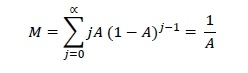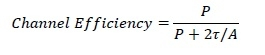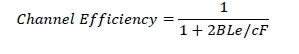# Ethernet Throughput

Throughput of a system refers to the rate of processing of a task thereby generating results. Ethernet is a set of technologies primarily used in LANs, whose primary data units are frames. The throughput of Ethernet is measured by the rate of successful delivery of frames over a communication channel.

There are several methods for representing Ethernet throughput. The least ambiguous among them is calculation of channel efficiency. Channel efficiency, is the percentage of the net bit rate (in bits per second) of a channel that is actually communicated. Suppose that an Ethernet connection has a speed of 100 Mbps. But, it is seen that effectively 80 Megabits of data are transmitted per second. Here, the channel efficiency is 80% or 0.8.

## Formulation of Channel Efficiency for Ethernet Throughput

Let us assume an Ethernet network has k stations and each station transmits with a probability p during a contention slot. Let A be the probability that some station acquires the channel. A is calculated as −

A = kp (1−p)kp

The value of A is maximized at p = 1/k. If there can be innumerable stations connected to the Ethernet network, i.e. k &arr; ∞, the maximum value of A will be 1/𝑒.

Let Q be the probability that the contention period has exactly j slots. Q is calculated as −

Q = A (1−A)j−1

Let M be the mean number of slots per contention. So, the value of M will be −Given that τ is the propagation time, each slot has duration 2τ. Hence the mean contention interval, 𝑤, will be 2τ/A.

Let P be the time is seconds for a frame to propagate.

The channel efficiency, when a number of stations want to send frame, can be calculated as:Let F be the length of frame, L be the cable length, c be the speed of signal propagation and 𝑒 be the contention slots per frame. The channel efficiency in terms of these parameters is −